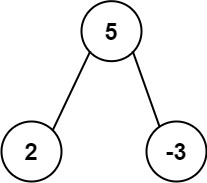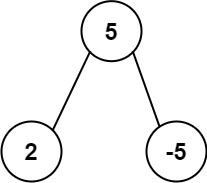# GeetCode Hub

Given the `root` of a binary tree, return the most frequent subtree sum. If there is a tie, return all the values with the highest frequency in any order.

The subtree sum of a node is defined as the sum of all the node values formed by the subtree rooted at that node (including the node itself).

Example 1:```Input: root = [5,2,-3]
Output: [2,-3,4]
```

Example 2:```Input: root = [5,2,-5]
Output: 
```

Constraints:

• The number of nodes in the tree is in the range `[1, 104]`.
• `-105 <= Node.val <= 105`

/** * Definition for a binary tree node. * public class TreeNode { * int val; * TreeNode left; * TreeNode right; * TreeNode() {} * TreeNode(int val) { this.val = val; } * TreeNode(int val, TreeNode left, TreeNode right) { * this.val = val; * this.left = left; * this.right = right; * } * } */ class Solution { public int[] findFrequentTreeSum(TreeNode root) { } }